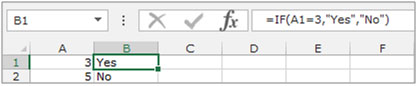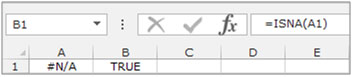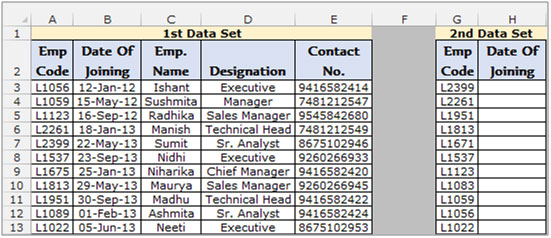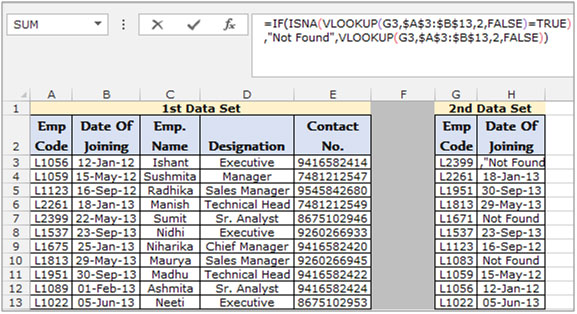ISNA function and how to use it to ignore the error in Vlookup formula

To eliminate the #N/A error while using the “VLOOKUP” function we need to use the “ISNA” function along with the “IF” function in Microsoft Excel 2010.

IF :-IF condition allows us to use multiple conditions in a cell. It helps to make the function flexible. . Excel allows up-to a maximum of 64 conditions which can be checked in a cell.
Syntax of “IF” function: =if (logical test, [value_if_true], [value_if_false])

Example:Cells A2 and A3 contain the numbers 3 and 5 respectively. Lets put the condition that if the number in the cell is 3 then the formula should display “Yes” otherwise “No”.

=IF (A1=3,”Yes”,”No”)ISNA: Returns the logical value “TRUE” if the formula returns the #N/A error otherwise it returns the value “FALSE”.

Syntax of “ISNA” function: =ISNA (value)To understand how you can get rid of the #N/A error in Microsoft Excel – lets check the below example

Example : We have 2 data sets in which we need to populate the joining date from the 1st data set into the 2nd data set.• Select the Cell H3, and write the formula.
• The function will return the joining date.
• Copy the formula to the rest of the cells to return the joining date for each row populated in column A.
• To Copy the formula in all cells press key “CTRL + C”in cell H3 and select the cellsH4 to H13 and press key “CTRL + V”on the keyboard.
• The employee codes which are present in the 2nd data set but not present in the 1st data set will return the “Not Found” result instead of the #N/A error.To conclude, you can use this formula if you want the formula to return some text instead of the #N/A or any such error. You can even ask the formula to return blank cells by replacing the “Not Found” with “”.

## Users are saying about us...

1.This is not a good formula because it uses four functions, and if the lookup value can be found, two VLOOKUPS are done, which is inefficient and calculation hungry when a large number of such formulae are deployed.
Besides, Excel 2007 introduced the IFERROR function which eliminates the need for IF function.
All that is required is: